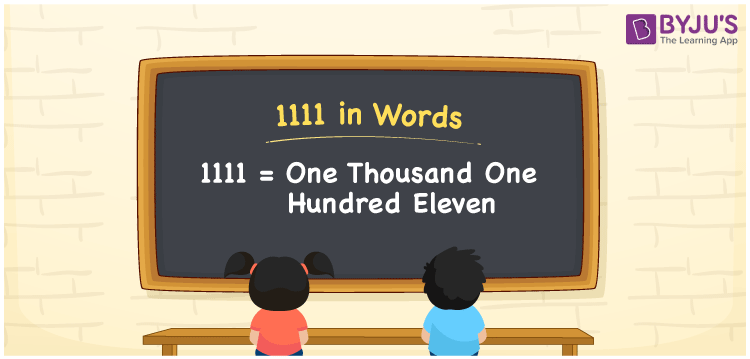# 1111 in Words

1111 in words is written as One thousand one hundred eleven. In both the International System of Numerals and the Indian System of Numerals, 1111 is written as One thousand one hundred eleven. The number 1111 is a Cardinal Number as it represents some quantity. For example, “the dress costs 1111 rupees.”

 1111 in words One thousand one hundred eleven One thousand one hundred eleven in Number 1111

## 1111 in English Words

1111 in English words is read as “One thousand one hundred eleven.”## How to Write 1111 in Words?

To write 1111 in words, we shall use the place value chart. In the place value chart, put 1 in the thousands, hundreds, tens and ones place respectively. Let us make a place value chart to write the number 1111 in words.

 Thousands Hundreds Tens Ones 1 1 1 1

Thus, we can write the expanded form as

1 × Thousand + 1 × Hundred + 1 × Ten + 1 × One

= 1 × 1000 + 1 × 100 + 1 × 10 + 1 × 1

= 1000 + 100 + 10 + 1

= 1111

= One thousand one hundred eleven.

1111 is a natural number, the successor of 1110 and the predecessor of 1112.

1111 in words – One thousand one hundred eleven

• Is 1111 an odd number? – Yes
• Is 1111 an even number? – No
• Is 1111 a perfect square number? – No
• Is 1111 a perfect cube number? – No
• Is 1111 a prime number? – No
• Is 1111 a composite number? – Yes

## Frequently Asked Questions on 1111 in Words

### How to write 1111 in words?

1111 in words is written as One thousand one hundred eleven.

### How to write 1111 in words in the International and Indian System of Numerals?

In both, the system of numerals, 1111 in words, is written as One thousand one hundred eleven.

### How to write 1111 in a place value chart?

In the place value chart, write 1 in the thousands, hundreds, tens and ones place respectively.